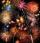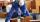# Unknown number

I think the number - its sixth is 3 smaller than its third.

Result

x =  18

#### Solution:

x/6 = x/3 - 3

x = 18

x = 18

Calculated by our simple equation calculator.

Leave us a comment of example and its solution (i.e. if it is still somewhat unclear...):Be the first to comment!#### To solve this verbal math problem are needed these knowledge from mathematics:

Need help calculate sum, simplify or multiply fractions? Try our fraction calculator. Do you have a linear equation or system of equations and looking for its solution? Or do you have quadratic equation?

## Next similar examples:

1. Unknown numberI think the number. I'll reduce it to its one-third. The result is then increased by one-third, and I get the number 12.
2. Unknown number 6Determine x if 1/6 of x is equal to 2/5 of the number 24.
3. Trees3/5 trees are apples, cherries are 1/3. 5 trees are pear. How many is the total number of trees?
4. Cleaning windowsCleaning company has to wash all the windows of the school. The first day washes one-sixth of the windows of the school, the next day three more windows than the first day and the remaining 18 windows washes on the third day. Calculate how many windows ha
5. ClassroomOne-eighth of 9th class was interested in studying at a grammar school, at a business academy one sixth, at secondary vocational schools quarter, to SOU one third and the remaining three students were interested in the school of art direction. How many st
6. One thirdIf 3/5 is 360, how much is 1/3?
7. EqnSolve equation with fractions: 2x/3-50=40+x/4
8. Simple equationSolve for x: 3(x + 2) = x - 18
9. PearsThere were pears in the basket, I took two-fifths of them, and left six in the basket. How many pears did I take?
10. EquationFind x: x + 1/2 = 1/3
11. Grandmother and grandfatherGrandmother baked cakes. Grandfather ate half, then quarter of the rest ate Peter and Paul ate half of rest. For parents left 6 cakes. How many cakes maked the grandmother?
12. Equation with fractionsSolve equation: ? It is equation with fractions.
13. King's birthdayTo celebrate the king's birthday workers fire 1/5 all purchased rockets. To celebrate the Queen's birthday fire 1/6 of the remaining rockets and to celebrate the birthday of king's son remaining 15,000 rockets. How many rockets they purchased?
14. Equation 15Solve equation with variables on both sides:
15. EquationSolve equation and check the result: 1.4x - 3/2 + x - 9,8 = x + 0,4/3 - 7 + 1,6/6
16. Bricklayers8 bricklayers build a house for 630 days. How many bricklayers have to add after 150 days, so then the whole building completed in (next) 320 days?
17. Equation with xSolve the following equation: 2x- (8x + 1) - (x + 2) / 5 = 9